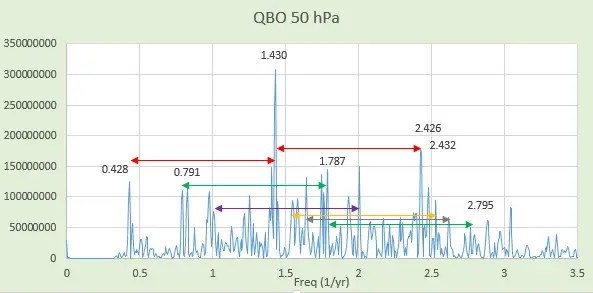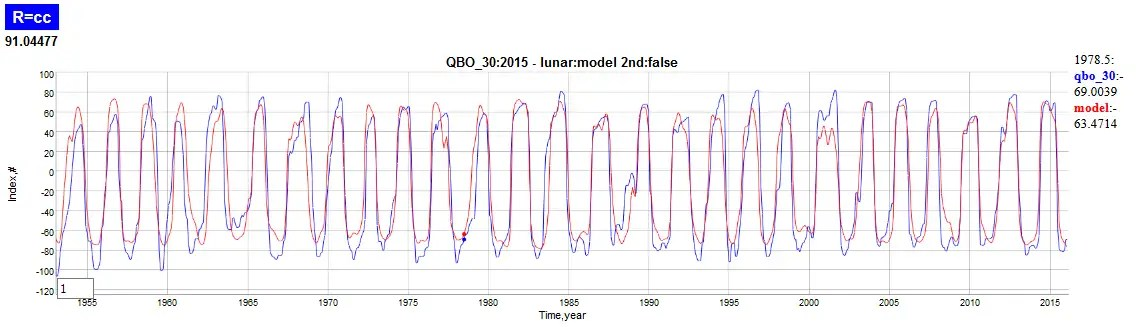# QBO Aliasing Graph

Before getting to the needless complexity discussion hinted at in the last post, here is a simple graphical analysis of finding aliased frequencies. Based on this analysis one can determine aliased signals by looking at the frequency power spectrum. The rules for aliasing in frequency space involve peeling back from the assumed frequency until the aliased harmonics appear in the spectrum.

1/T = 1/P + n, where P is the tidal period in years, and n is … -3, -2, -1, 0

So applying the Draconic period aliasing to QBO, the harmonics are clearly seen in the following Figure 1.  The strongest factor is the approximately 2.33 year period shown on the left, but since this is a second derivative of the QBO time series, higher frequency aliased harmonics are also observed to the right.  Note that the factors are approximately 0.43, 1.43, and 2.43, which are separated by Δn=1. And note that 1/0.43 ~ 2.33 years, which is the approximate observed QBO frequency..Fig. 1:  Spectrum of the second derivative of QBO, showing seasonally aliased factors

Other potential periods are shown in the figure, including the unaliased annual and semi-annual signals separated by the violet colored arrow. The green arrow separates the harmonics of the 13.633 day lunar tide periodicity. Clustered around the strong Draconic period are also satellite frequencies. At least one of these satellites along with the strong aliased Draconic also appear in the LOD spectrum. That provides the physical basis for QBO (and also ENSO) forcing.

Putting these factors together via multiple regression results in marvelous fits of the various QBO time series, as seen in Figure 2 below.Fig. 2:  Multiple regression fit of the 30 hPa QBO time series.

And to highlight the second derivative composition and how the higher frequency aliased harmonics contribute to the fine detail, see Figure 3.Fig. 3 : Second derivative of QBO highlights the higher frequency aliased harmonic detail.

Only in a few places do the aliased harmonics not appear well aligned. Those years, such as the interval 1983-1984 may be telling us that other climate events may be playing a role in temporarily impacting the QBO dynamics.

## 4 thoughts on “QBO Aliasing Graph”

1.admin

Same thing with ENSO. All these are aliased, with the ones sharing the same symbol shifted by 2pi in the frequency spectrum.

This is the model fit FFT.

Like

2. Pingback: Needless Complexity | context/Earth